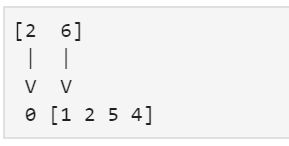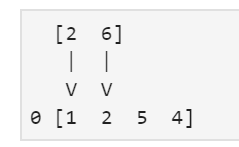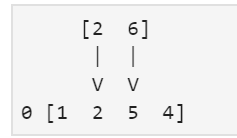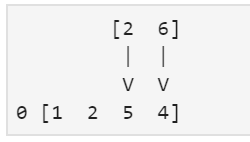# Types of padding in convolution layer

Let’s discuss padding and its types in convolution layers. In convolution layer we have kernels and to make the final filter more informative we use padding in image matrix or any kind of input array. We have three types of padding that are as follows.

Let’s assume a kernel as a sliding window. We have to come with the solution of padding zeros on the input array. This is a very famous implementation and will be easier to show how it works with a simple example, consider x as a filter and h as an input array.

x[i] = [6, 2]
h[i] = [1, 2, 5, 4]

Using the zero padding, we can calculate the convolution.

You have to invert the filter x, otherwise the operation would be cross-correlation. First step, (now with zero padding):= 2 * 0 + 6 * 1 = 6

Second step:= 2 * 1 + 6 * 2 = 14

Third step:= 2 * 2 + 6 * 5 = 34

Fourth step:= 2 * 5 + 6 * 4 = 34

Fifth step:

= 2 * 4 + 6 * 0 = 8

The result of the convolution for this case, listing all the steps above, would be: Y = [6 14 34 34 8]

 `# importing numpy ` `import` `numpy as np ` ` `  `x ``=` `[``6``, ``2``] ` `h ``=` `[``1``, ``2``, ``5``, ``4``] ` ` `  `y ``=` `np.convolve(x, h, ``"full"``) ` `print``(y)   `

Output:

```[ 6 14 34 34  8]
```

In this type of padding, we only append zero to the left of the array and to the top of the 2D input matrix.

 `# importing numpy ` `import` `numpy as np ` ` `  `x ``=` `[``6``, ``2``] ` `h ``=` `[``1``, ``2``, ``5``, ``4``] ` ` `  `y ``=` `np.convolve(x, h, ``"same"``) ` `print``(y) `

Output:

```[ 6 14 34 34]
```

In this type of padding, we got the reduced output matrix as the size of the output array is reduced. We only applied the kernel when we had a compatible position on the h array, in some cases you want a dimensionality reduction.

 `# importing numpy ` `import` `numpy as np ` ` `  `x ``=` `[``6``, ``2``] ` `h ``=` `[``1``, ``2``, ``5``, ``4``] ` ` `  `y ``=` `np.convolve(x, h, ``"valid"``) ` `print``(y) `

Output:

```[14 34 34]
```

My Personal Notes arrow_drop_upCheck out this Author's contributed articles.

If you like GeeksforGeeks and would like to contribute, you can also write an article using contribute.geeksforgeeks.org or mail your article to contribute@geeksforgeeks.org. See your article appearing on the GeeksforGeeks main page and help other Geeks.

Please Improve this article if you find anything incorrect by clicking on the "Improve Article" button below.

Article Tags :

1

Please write to us at contribute@geeksforgeeks.org to report any issue with the above content.